# What are Numbers

Go back to  'Numbers'

 1 What is a Number? 2 Types of Numbers and Definitions 3 Even and Odd Numbers 4 Numeric Numbers 5 Natural and Whole Numbers 6 Important Notes on Numbers 7 Solved Examples of Numbers 8 Challenging Questions on Numbers 9 Practice Questions on Numbers 10 Think Tank 11 Maths Olympiad Sample Papers 12 Frequently Asked Questions(FAQs)

We at Cuemath believe that Math is a life skill. Our Math Experts focus on the “Why” behind the “What.” Students can explore from a huge range of interactive worksheets, visuals, simulations, practice tests, and more to understand a concept in depth.

Book a FREE trial class today! and experience Cuemath’s LIVE Online Class with your child.

## What is a Number?

What do you notice when you see a group of objects like toys, a book, pencils etc.?

We can notice the number of objects, their colours, their sizes and shapes and so on.

If you are asked to count these objects, how would you do that?

We can use numbers to count these objects.

So, what are numbers?

Let's learn the meaning of the word "numbers" first.

### Number: Definition

A number is a mathematical representation of counting and measuring objects.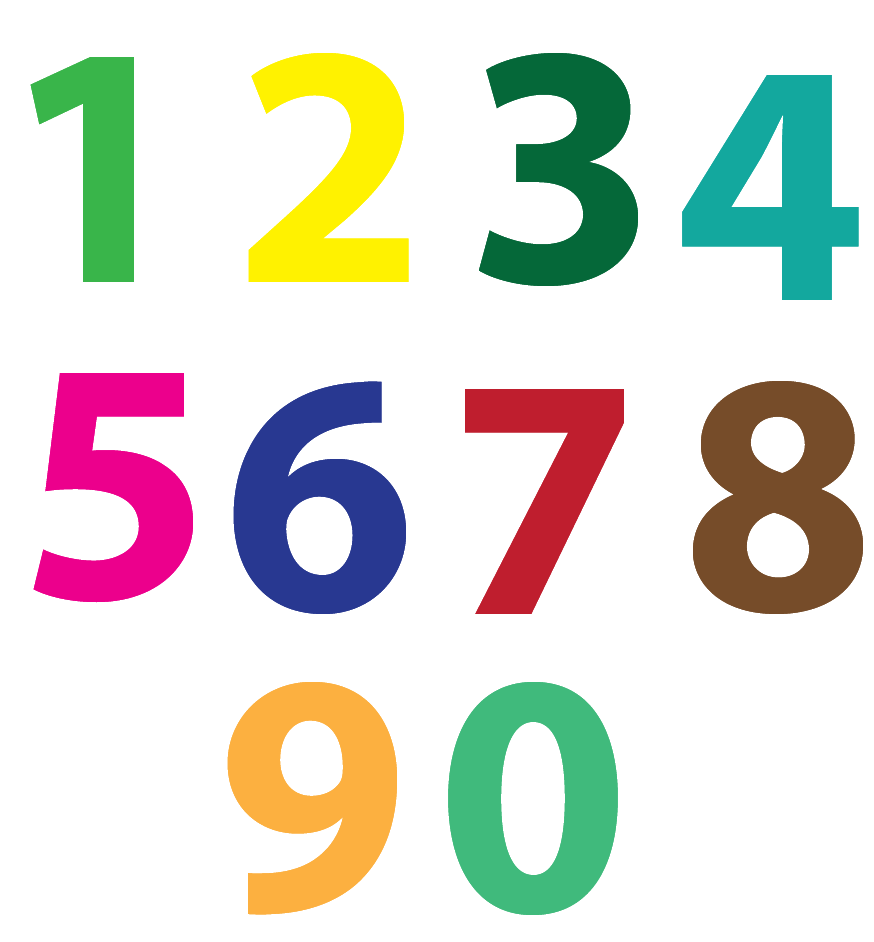Do you know how many numbers are there?

What is the biggest number you are aware of?

Are there any limitations to numbers?

You will find answers to these questions by the end of this topic.

To answer the question about how many numbers are there, you should know that there are infinite numbers in Maths.

You can compare numbers to the infinite number of stars in the sky.

So, why do we need numbers?

We need numbers to represent our age, date of birth, class, height, weight, time, marks, rank, etc.

Numbers are very important and we tend to use them throughout our lives.

Let us now learn about the various types of numbers.

## Types of Numbers and Definitions

There are many different types of numbers present in Maths.

They are:

### 1. Natural numbers

All numbers from 1 to infinity (countless) are called natural numbers.

### 2. Whole numbers

The numbers which start from zero (0) are called whole numbers.

In other words, the combination of zero and natural numbers are called whole numbers.

### 3. Integers

The positive and negative numbers along with zero are called integers.

Zero does not stand for positive or negative.

It is neutral in the centre.

The positive numbers are called positive integers and negative numbers are called negative integers.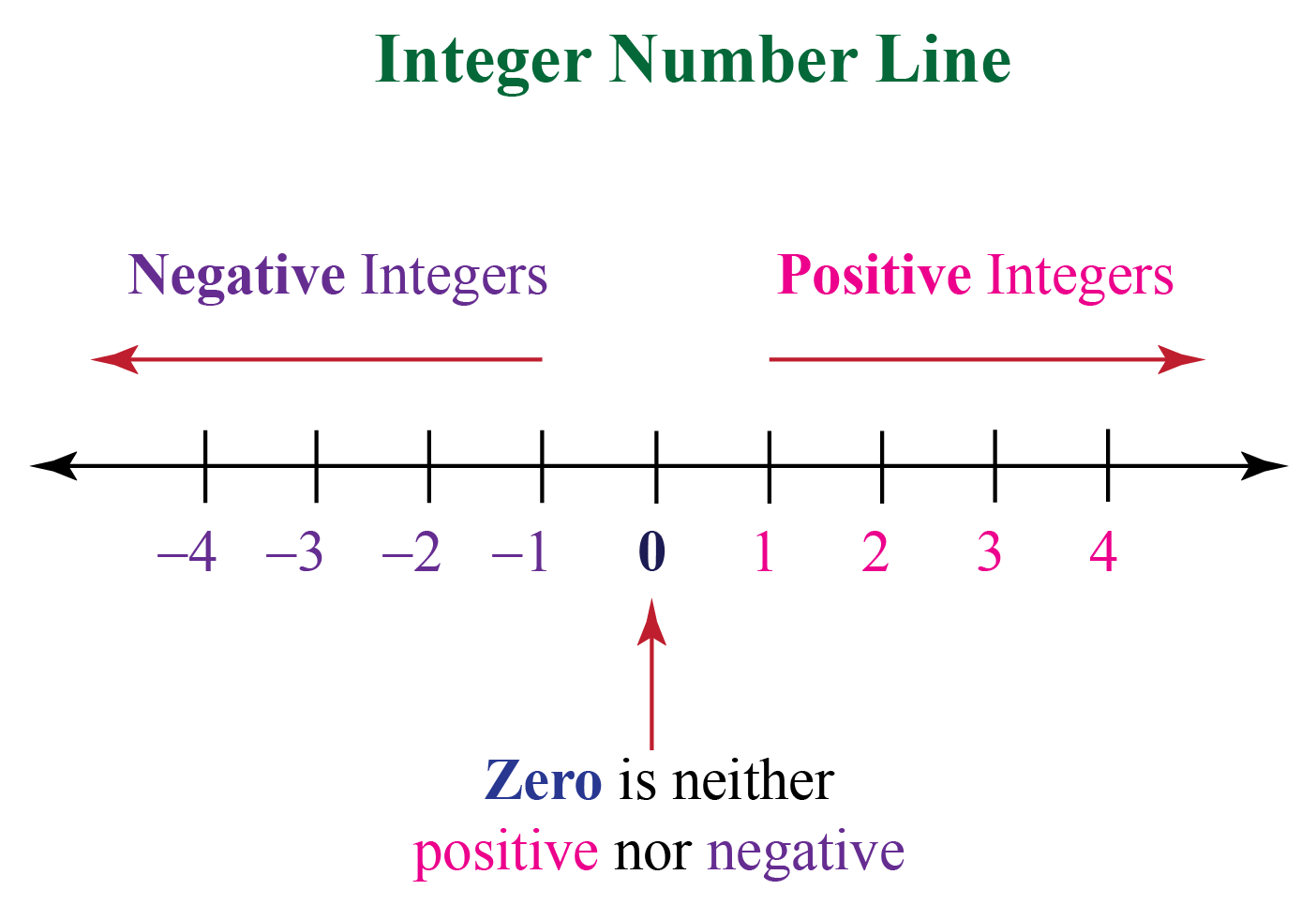### 4. Rational numbers

Any number which is defined in the form of a fraction or ratio is called a rational number.

This may consist of the numerator (p) and denominator (q).

A rational number can be a whole number or an integer.

Example 1: Divide $\begin{equation}\frac{2}{3}\end{equation}$

$\begin{equation}\frac{2}{3}=0.6666=0.67\end{equation}$

The decimal value is recurring (repeating), so we approximate it to 0.67

Example 2: Find $\begin{equation}\sqrt{4}\end{equation}$

$\begin{equation}\sqrt{4}=2\end{equation}$

The answer is a whole number.

### 5. Irrational numbers

Irrational numbers are those in which the fraction or the ratio cannot be determined i.e. it cannot be expressed in the form of a fraction or a ratio.

Irrational numbers cannot be expressed in decimal form since the decimal numbers extend continuously and never repeat.

For instance,  $$\pi$$ (pi) is an irrational number because it does not have any decimal pattern and it go without an end.

Example 1: Find the value of $$\pi$$

\begin{align}\pi=\frac{22}{7}\end{align}

\begin{align}\frac{22}{7}=3 \cdot 142857\dots\end{align}

The decimal value in non-recurring and goes on without an end.

\begin{align}\sqrt{2}=1 \cdot 414213\dots\end{align}

\begin{align}e=2\cdot 718281\dots\end{align}

\begin{align}\sqrt{3}=1 \cdot 73205\dots\end{align}

These are some examples of irrational numbers.

### 6. Real numbers

Real numbers in maths are the numbers which includes both rational and irrational numbers.

Therefore, it includes natural numbers, whole numbers and integers.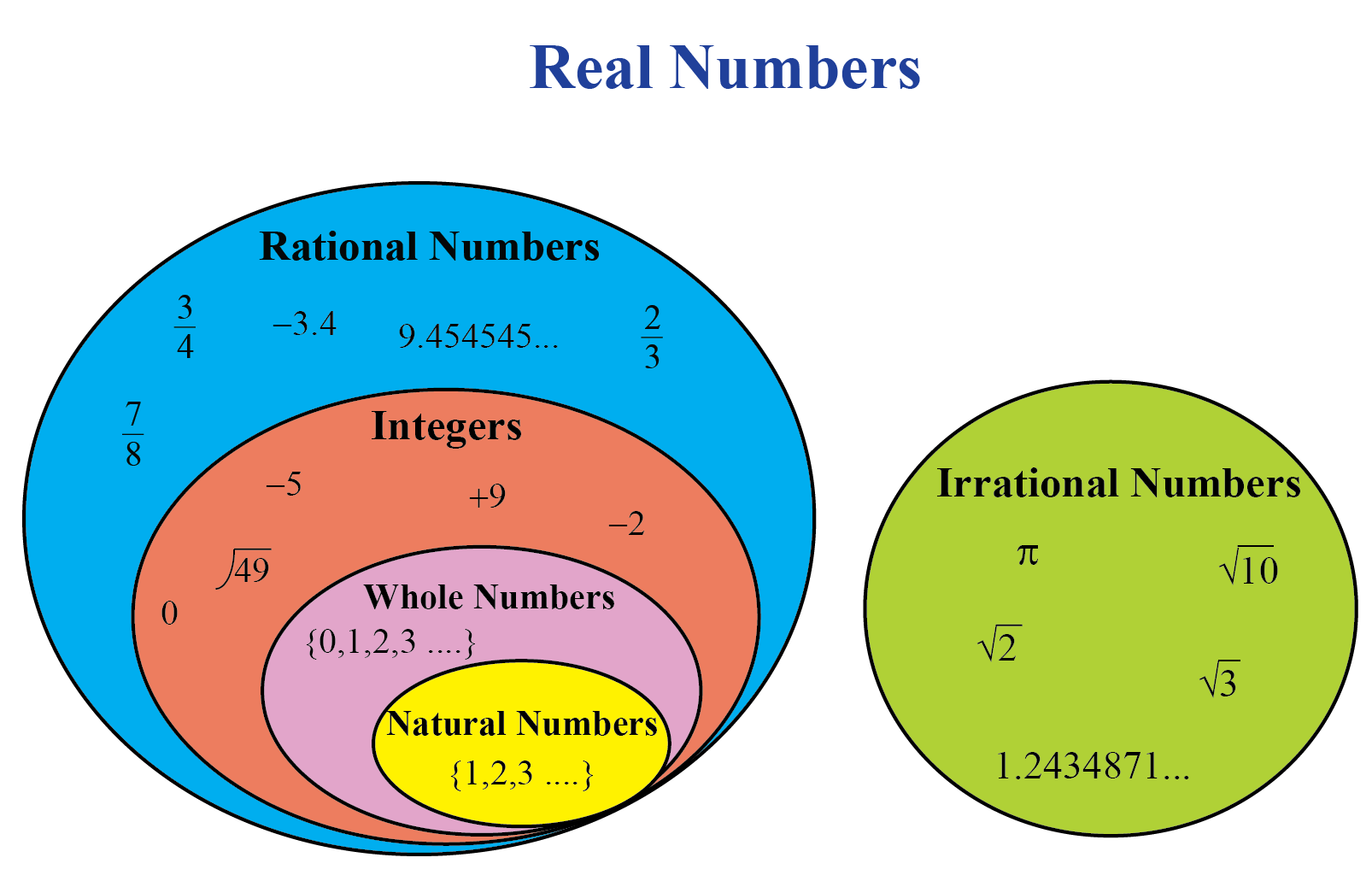### 7. Imaginary numbers

An imaginary number is a number which is represented as any real number multiplied with the imaginary unit "i" where "i" has some value.

\begin{align}i =\sqrt{-1}\end{align}

\begin{align}i^{2}=-1\end{align}

\begin{align}i^{3}=-i=-\sqrt{-1}\end{align}

\begin{align}i^{4}=1\end{align}

These are some of the imaginary numbers.

### 8. Complex numbers

Complex numbers are the combination of both real and imaginary numbers in the form of an equation.

It can be expressed in the form of x+iy where x and y are real numbers and i is the imaginary number.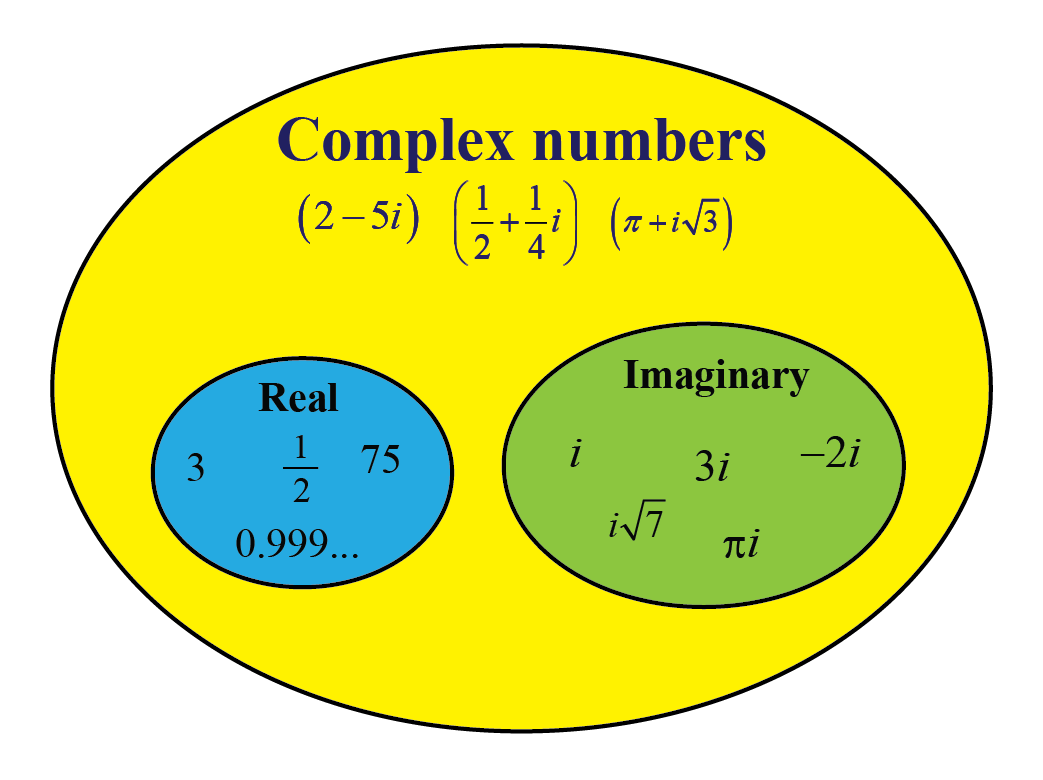Thus, these are the different types of numbers in Mathematics.

## Even and Odd numbers

### Even number

Any number that ends with 0, 2, 4, 6 and 8 and is divisible by 2 is called an even number.

The number which is divisible by 2 and gives zero as the remainder is called an even number.

### Odd number

Any number which is not divisible by 2 is called an odd number.

An odd number always gives one as a remainder when it is divided by 2.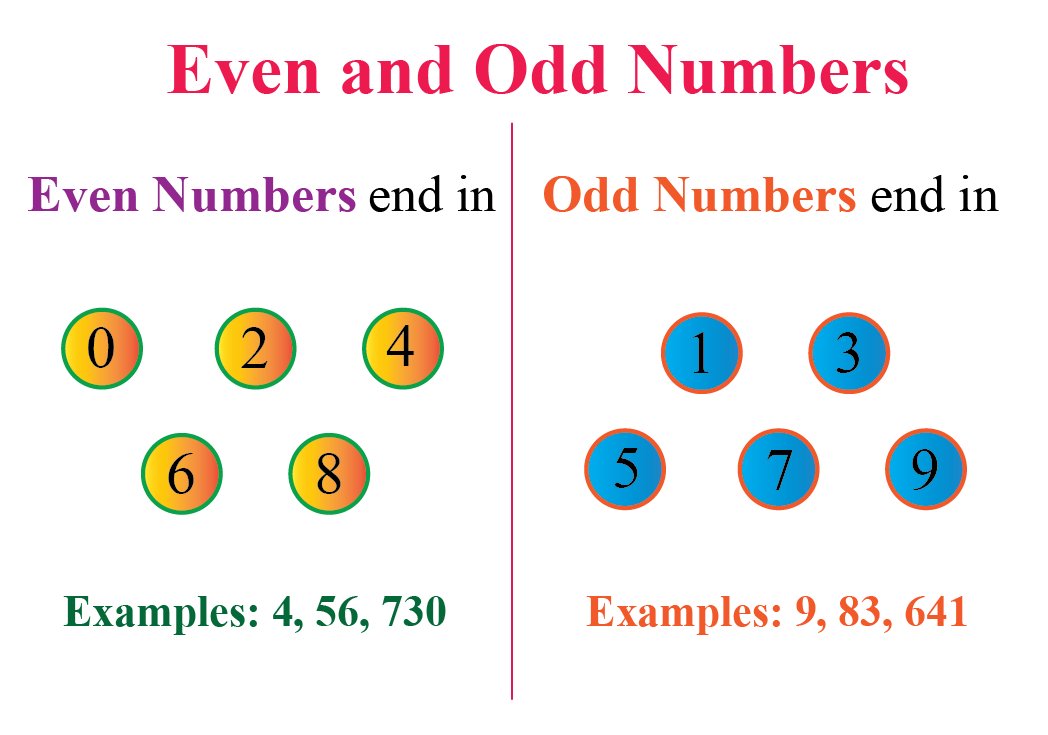## Numeric Numbers

The word "Numeric" refers to anything that contains numbers.

The numeric numbers are represented in the form of digits from (0 to 9)

Ex: The number "23" is represented by two digits "2" and "3" and not in the form of words(twenty-three).

There is also a combination of alphabet and numbers which is called "Alphanumeric".

Alphanumeric consists of the alphabet (A-Z, a-z), digits (0-9) and symbols(@, #, &)

## Natural and Whole numbers

### Natural numbers

The numbers from 1 to infinity (countless) are called natural numbers.

The number 1 is the smallest natural number.

Natural numbers are generally used for basic counting and sorting objects.

 N = {1,2,3,4,.....n}

### Whole numbers

Before moving onto whole numbers, let's first discuss about "Zero" (0)

What is Zero?

Zero is a numerical digit in Maths.

An interesting fact is that the word "zero" means "nothing" or "absence" but the number zero plays an important role in the numerical system.

This number zero when placed on the right of any digit forms a different number or value.

The numbers which start from zero (0) are called whole numbers.

In other words, the combination of zero and natural numbers are called whole numbers.

The number zero is the smallest whole number.

 W = {0,1,2,3,4,5...}

Help your child score higher with Cuemath’s proprietary FREE Diagnostic Test. Get access to detailed reports, customised learning plans and a FREE counselling session. Attempt the test now.

## Solved Examples on Numbers

 Example 1

Rohan and Riya are playing with number cards.

They want to classify the numbers based on their types.

Help Rohan and Riya complete their game.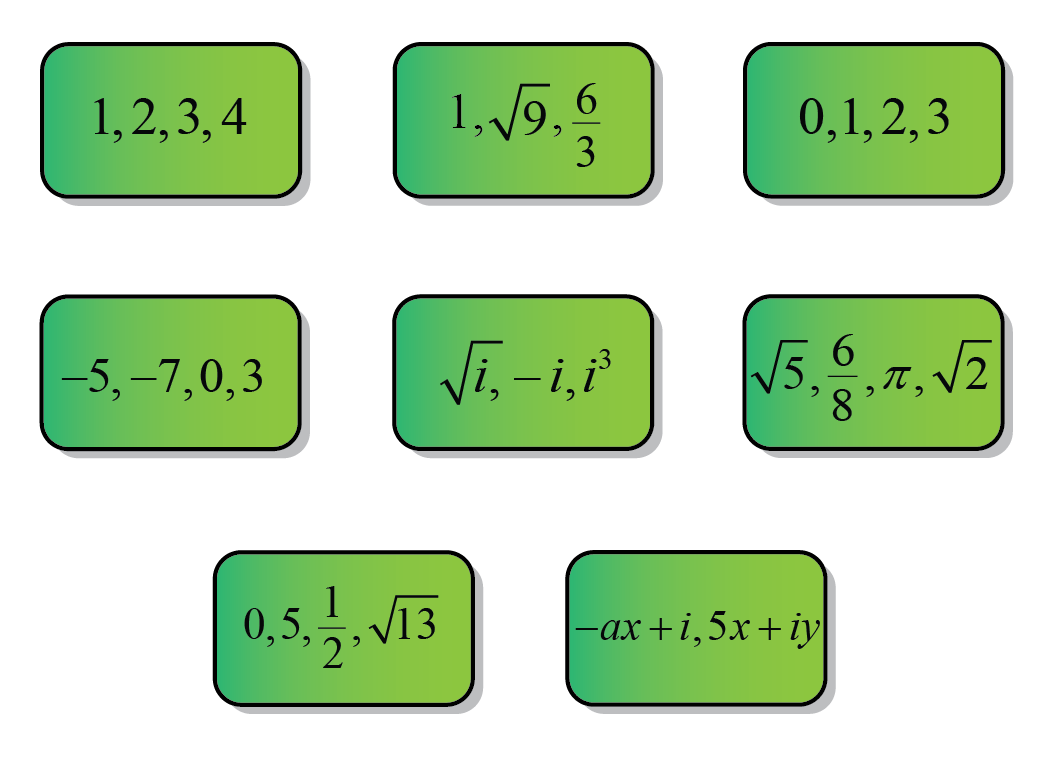Solution: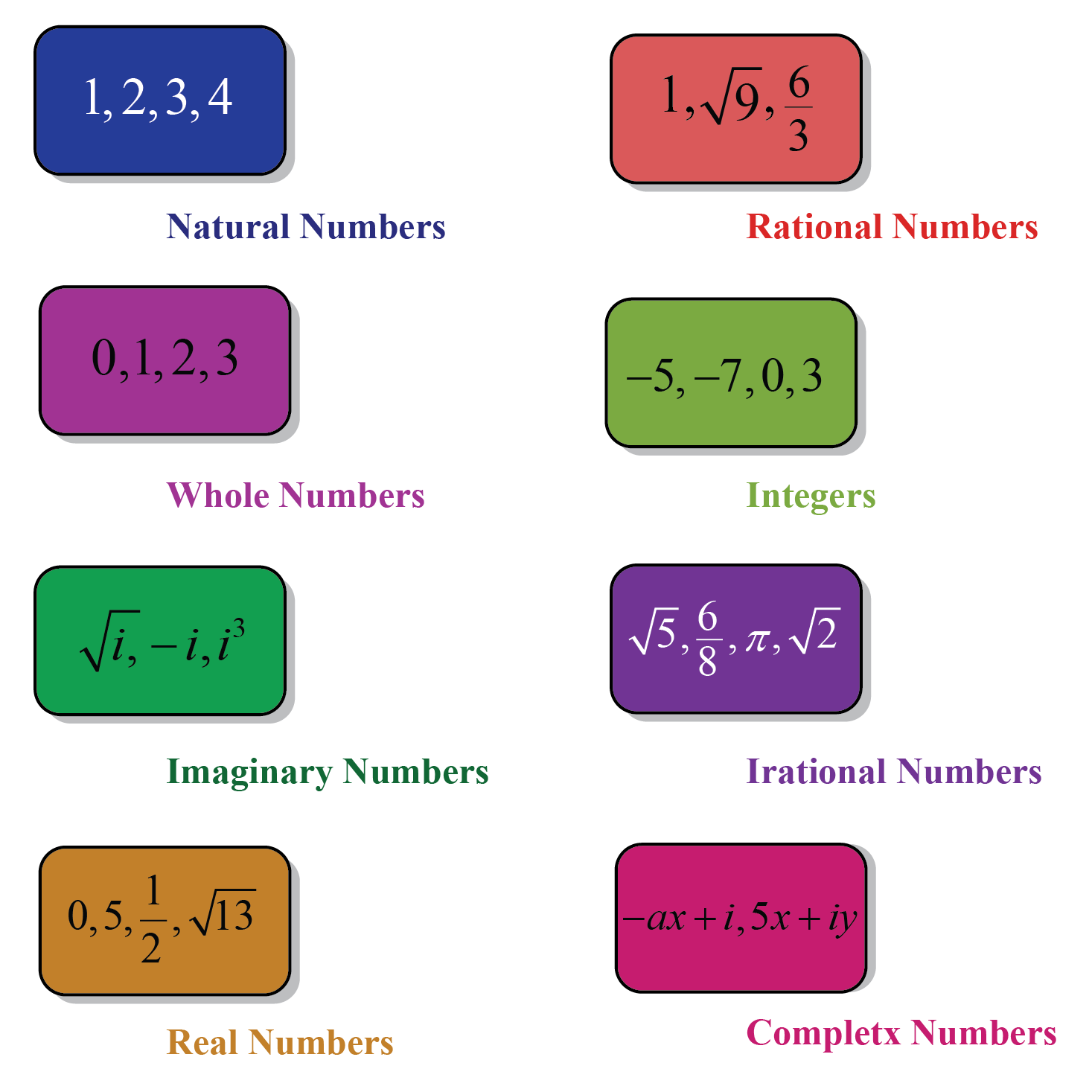$$\therefore$$ The numbers are classified.
 Example 2

Rohan and Tina were given a Math challenge.

They were asked to check if \begin{align}\frac{1}{4}\end{align} is rational or irrational?

Rohan says it is rational.

Tina disagrees. She says it is an irrational number.

Find out who won this challenge.

Solution:

$$\begin{equation}\frac{1}{4}= 0.25\end{equation}$$

From the above, it is clear that the decimal value terminates at one point.

Therefore, $$\begin{equation}\frac{1}{4}\end{equation}$$ is a rational number.

 $$\therefore$$Rohan won the challenge.
 Example 3

Ram is learning about rational and irrational numbers.

Help Ram check whether $$\begin{equation}\sqrt{5}\end{equation}$$ is rational or irrational.

Solution:

$\begin{equation}\sqrt{5}=2.23606797749979\end{equation}$

From the above, it is clear that the decimal point is neither recurring nor terminating at any point.

Therefore, the given number is an irrational number.

 $$\therefore$$ $$\sqrt{5}$$ is an irrational number.
 Example 4

Rohan has a bag of papers with the following numbers written on them.

\begin{align}\sqrt{7}, 5, 9.5 , -11, 2, 3 , 6i \end{align}

He was asked to pick only natural numbers from the bag.

Help Rohan find all the natural numbers from the bag.

Solution:

The natural numbers from the bag are 5, 2 and 3

 $$\therefore$$ Rohan should pick 5, 2, and 3Challenging Questions
1. Is number 0 rational or irrational?

CLUEless in Math? Check out how CUEMATH Teachers will explain What are Numbers to your kid using interactive simulations & worksheets so they never have to memorise anything in Math again!

Explore Cuemath Live, Interactive & Personalised Online Classes to make your kid a Math Expert. Book a FREE trial class today!

## Practice Questions on NumbersThink Tank
1. Which number cannot be expressed as a Roman numeral?
2. Which number is called "the magic number"?

Hint: The number is between 0 to 9

IMO (International Maths Olympiad) is a competitive exam in Mathematics conducted annually for school students. It encourages children to develop their math solving skills from a competition perspective.

## 1. Is the number 0 a number?

Yes, zero '0' is also a number and it is the smallest whole number.

## 2. What are the first ten numbers?

The first ten numbers are 0,1,2,3,4,5,6,7,8,9

## 3. What are the types of numbers?

The various types of numbers are:

• natural
• whole
• integer
• rational
• irrational
• real
• imaginary and
• complex

## 4. What is a number? Explain.

A number is a mathematical representation of counting and measuring objects.

We use numbers to represent our age, date of birth, class, height, weight, time, marks, ranks, etc.

More Important Topics
Numbers
Algebra
Geometry
Measurement
Money
Data
Trigonometry
Calculus